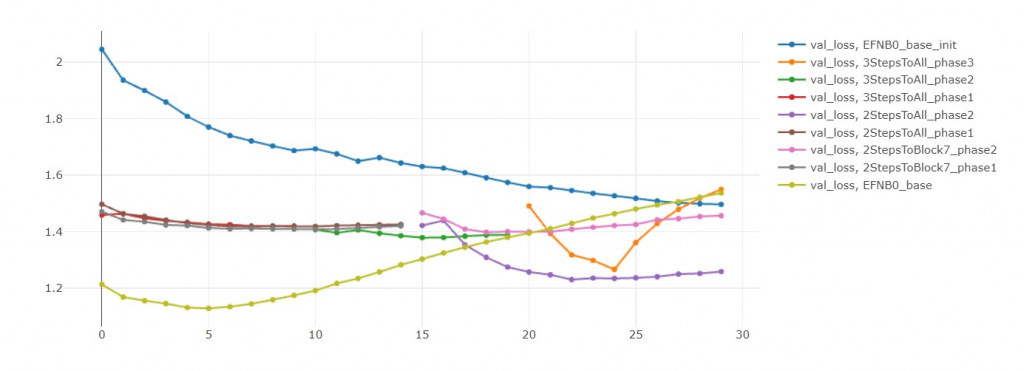DAY 20
0
AI & Data

前言

1. 解決資料不平衡(Done)
2. 學習率的設定(Done)
3. 訓練輪數(Nice to have)
4. 模型深度(No, I want my model to be more efficient ! )
5. 階段式訓練(Now)

訓練輪數(epoch)

(如果資料量很大，建議不要忍一下，直接買Pro方案)遷移學習

遷移學習三方法

3. Fine-tune

Fine-tune會多訓練幾層。(通常是靠近輸出層的網路層)

1. 解凍最後一層，訓練10輪
2. 解凍最後兩層，再訓練10輪
3. 解凍最後三層，再訓練10輪
這樣模型才會穩定的收斂，
因為(在有些仍凍結的情況下)一次微調太多層的參數很容易不收斂，
有可能還不如把全部預訓練參數都進行微調。

程式碼

建立一個凍結參數的預訓練模型

def build_model(preModel=EfficientNetB0,
pretrained=True,
num_classes=7,
input_shape=(48, 48, 3),
l2_coef=0.0001):

pre_model = preModel(include_top=False, weights='imagenet' if pretrained == True else None,
input_shape=input_shape,
pooling='max', classifier_activation='softmax')

for layer in pre_model.layers:
layer.trainable = False

x = Dropout(0.2)(pre_model.output)

output = Dense(
num_classes, activation="softmax", name="main_output",
kernel_regularizer=regularizers.l2(l2_coef))(x)

freezed_model = tf.keras.Model(pre_model.input, output)

loss=tf.keras.losses.CategoricalCrossentropy(),
metrics=['accuracy'])

return freezed_model

如何解開凍結的參數

def unfreeze_model(model, n=1, is_unfreeze_BN=False):
# We unfreeze the top n layers while leaving BatchNorm layers frozen
# n = 6 (~ block-top)
# n = 19 (~ block-7)
# n = 78 (~ block-6)
if is_unfreeze_BN == False:
for layer in model.layers[-n:]:
if not isinstance(layer, BatchNormalization):
layer.trainable = True
else:
for layer in model.layers[-n:]:
layer.trainable = True

如何做二階段式訓練

• 6和19不是隨便選的數字，這兩個數字分別是EfficinetNetB0的最後兩個Block的網路層
unfreeze_n = [6, 19]
phase_epochs = [15, 30]
phase_batch_size = [32, 32]
phases = len(phase_epochs)

model = build_model()
for i in range(phases):
unfreeze_model(model, n=unfreeze_n[i])
model.fit(X_train, y_train_oh,
validation_data=(X_val, y_val_oh),
initial_epoch=0 if i == 0 else phase_epochs[i-1],
epochs=phase_epochs[i],
batch_size=phase_batch_size[i])

實驗結果

Block-top 6
Block-7 19
Block-6 78
Block-all 全部

初始模型 vs 模型零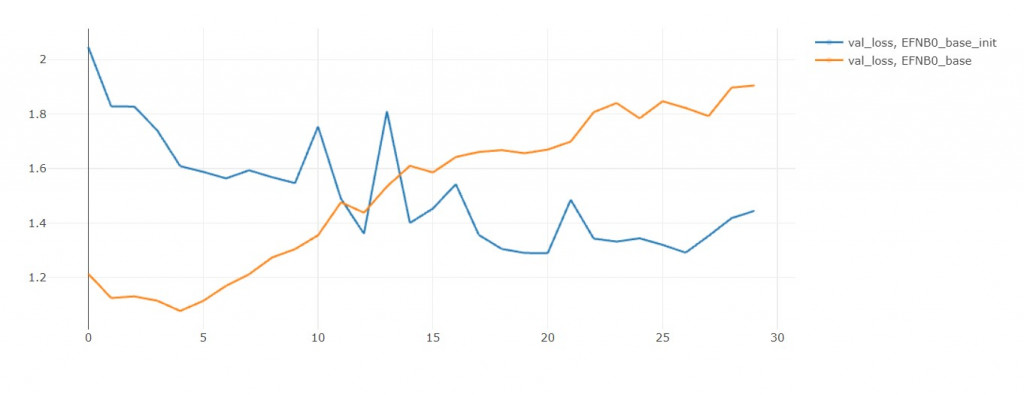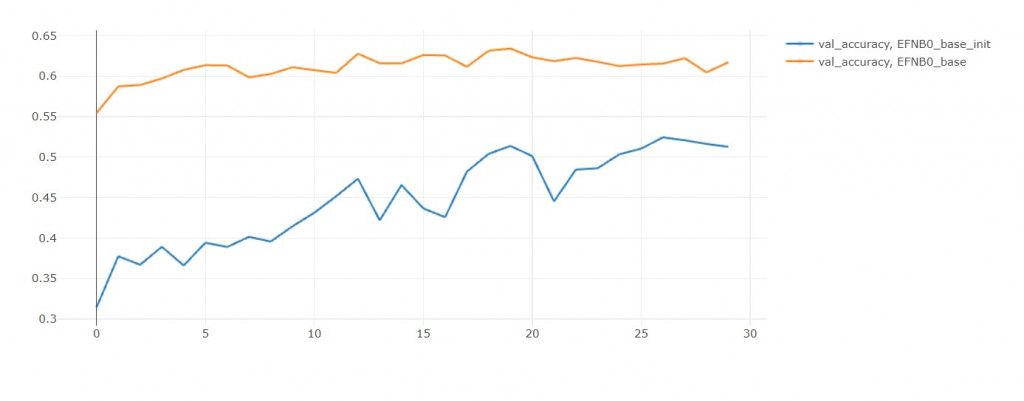模型一 vs 模型二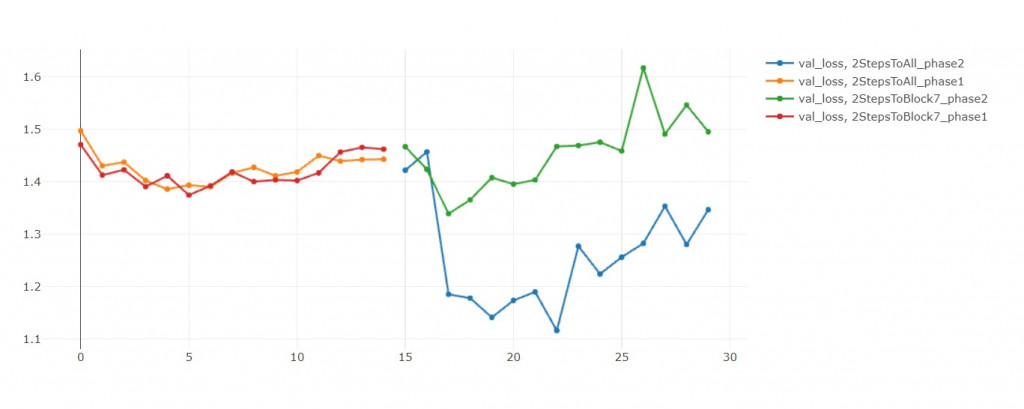模型二 vs 模型三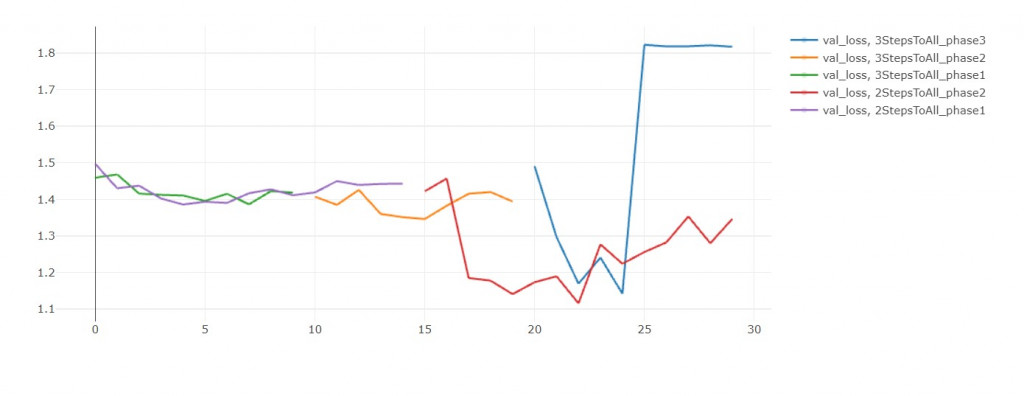結語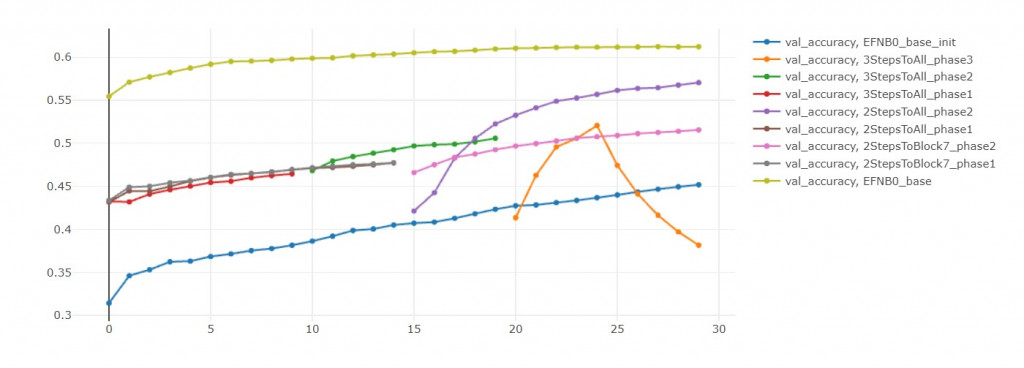EFN_base的損失值簡直快要飛上天和太陽肩並肩 = =